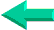This is an excerpt from Pierre Leclerc (www.excel-vba.com)

The 2 Most Important

Excel Functions AND
Formulas

SUMPRODUCT

AND

INDEX/MATCH

## Annex 9: Excel Spreadsheets Statistical Functions (83)

There are 83 functions in the "Statistical" category.

 Functions What it Does AVERAGE Returns the average of its arguments COUNT Counts how many numbers are in the list of arguments AVERAGEA Returns the average of its arguments, including numbers, text, and logical values COUNTA Counts how many values are in the list of arguments) RANK Returns the rank of a number in a list of numbers LARGE Returns the k-th largest value in a data set SMALL Returns the k-th smallest value in a data set COUNTBLANK Counts the number of blank cells within a range COUNTIF Counts the number of nonblank cells within a range that meet the given criteria (SUMPRODUCT does better) AVEDEV Returns the average of the absolute deviations of data points from their mean BETADIST Returns the cumulative beta probability density function BETAINV Returns the inverse of the cumulative beta probability density function BINOMDIST Returns the individual term binomial distribution probability CHIDIST Returns the one-tailed probability of the chi-squared distribution CHIINV Returns the inverse of the one-tailed probability of the chi-squared distribution CHITEST Returns the test for independence CONFIDENCE Returns the confidence interval for a population mean CORREL Returns the correlation coefficient between two data sets COVAR Returns covariance, the average of the products of paired deviations CRITBINOM Returns the smallest value for which the cumulative binomial distribution is less than or equal to a criterion value DEVSQ Returns the sum of squares of deviations EXPONDIST Returns the exponential distribution FDIST Returns the F probability distribution FINV Returns the inverse of the F probability distribution FISHER Returns the Fisher transformation FISHERINV Returns the inverse of the Fisher transformation FORECAST Returns a value along a linear trend FREQUENCY Returns a frequency distribution as a vertical array FTEST Returns the result of an F-test GAMMADIST Returns the gamma distribution GAMMAINV Returns the inverse of the gamma cumulative distribution GAMMALN Returns the natural logarithm of the gamma function, Γ (x) GEOMEAN Returns the geometric mean GROWTH Returns values along an exponential trend HARMEAN Returns the harmonic mean HYPGEOMDIST Returns the hypergeometric distribution INTERCEPT Returns the intercept of the linear regression line KURT Returns the kurtosis of a data set LINEST Returns the parameters of a linear trend LOGEST Returns the parameters of an exponential trend LOGINV Returns the inverse of the lognormal distribution LOGNORMDIST Returns the cumulative lognormal distribution MAX Returns the maximum value of a range MAXA Returns the maximum numerical value of a range that includes numerical and alphabetical values MEDIAN Returns the median of the given numbers MIN Returns the minimum value of a range MINA Returns the minimum numerical value of a range that includes numerical and alphabetical values MODE Returns the most common value in a data set NEGBINOMDIST Returns the negative binomial distribution NORMDIST Returns the normal cumulative distribution NORMINV Returns the inverse of the normal cumulative distribution NORMSDIST Returns the standard normal cumulative distribution NORMSINV Returns the inverse of the standard normal cumulative distribution PEARSON Returns the Pearson product moment correlation coefficient PERCENTILE Returns the k-th percentile of values in a range PERCENTRANK Returns the percentage rank of a value in a data set PERMUT Returns the number of permutations for a given number of objects POISSON Returns the Poisson distribution PROB Returns the probability that values in a range are between two limits QUARTILE Returns the quartile of a data set RSQ Returns the square of the Pearson product moment correlation coefficient SKEW Returns the skewness of a distribution SLOPE Returns the slope of the linear regression line SMALL Returns the k-th smallest value in a data set STANDARDIZE Returns a normalized value STDEV Estimates standard deviation based on a sample STDEVA Estimates standard deviation based on a sample, including numbers, text, and logical values STDEVP Calculates standard deviation based on the entire population STDEVPA Calculates standard deviation based on the entire population, including numbers, text, and logical values STEYX Returns the standard error of the predicted y-value for each x in the regression TDIST Returns the Student's t-distribution TINV Returns the inverse of the Student's t-distribution TREND Returns values along a linear trend TRIMMEAN Returns the mean of the interior of a data set TTEST Returns the probability associated with a Student's t-test VAR Estimates variance based on a sample VARA Estimates variance based on a sample, including numbers, text, and logical values VARP Calculates variance based on the entire population VARPA Calculates variance based on the entire population, including numbers, text, and logical values WEIBULL Returns the Weibull distribution ZTEST Returns the two-tailed P-value of a z-test New Functions in Excel 2007 AVERAGEIF Calculates the average within a range that meet a given criteria (SUMPRODUCT does better) AVERAGEIFS Calculates the average within a range that meet one or many given criteria (SUMPRODUCT does better) COUNTIFS Counts the number of nonblank cells within a range that meet the given criteria (SUMPRODUCT does better)

SUMPRODUCT  ¦  INDEX/MATCH  ¦  SUBTOTAL  ¦  ISERROR  ¦  HLOOKUP  ¦  LOOKUP  ¦  VLOOKUP
AVERAGEIF  ¦  AVERAGEIFS ¦  COUNTIF  ¦  COUNTIFS  ¦  SUMIF  ¦  SUMIFS  ¦  IFERRORBack home